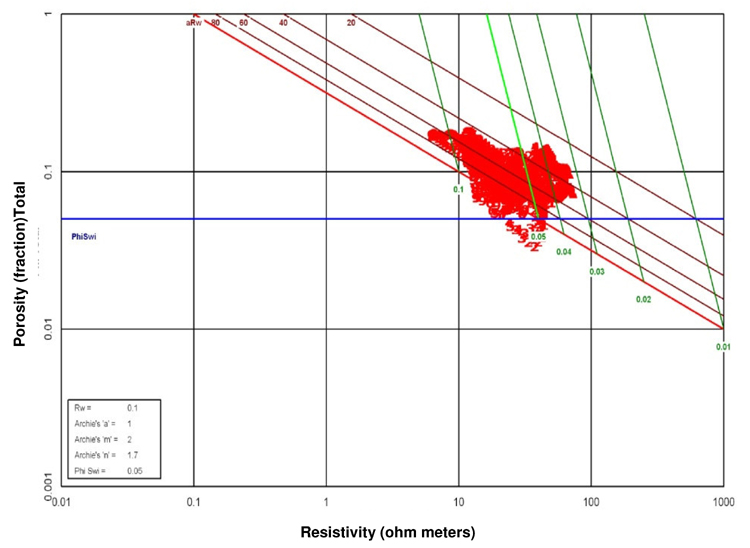Figure 3. Interpretation of porosity vs. resistivity relations to determine parameters for Archie analysis. a (cementation constant) = 1.0, Rw (water resistivity) = 0.1, m (cementation exponent) = 2, and n (saturation exponent) = 1.7.